# Gases Boyles Law Boyles Law As the volume

• Slides: 45Gases Boyle’s LawBoyle’s Law • As the volume of a gas increases, the pressure decreases. – Temperature remains constant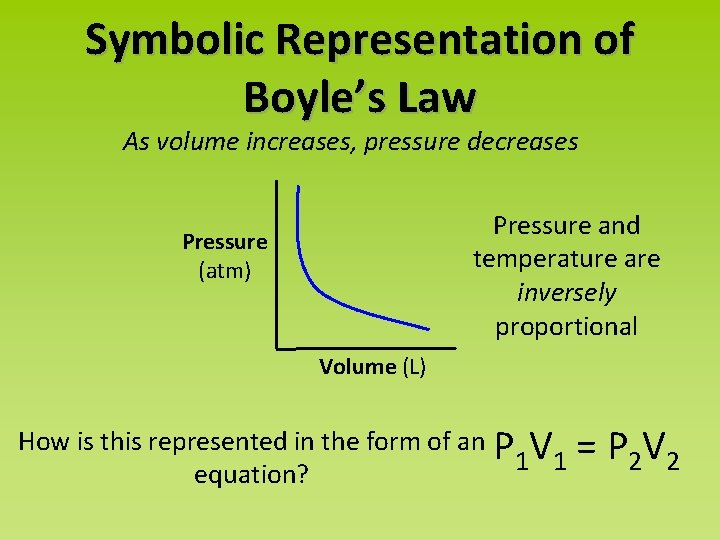Symbolic Representation of Boyle’s Law As volume increases, pressure decreases Pressure and temperature are inversely proportional Pressure (atm) Volume (L) How is this represented in the form of an P V 1 1 equation? = P 2 V 2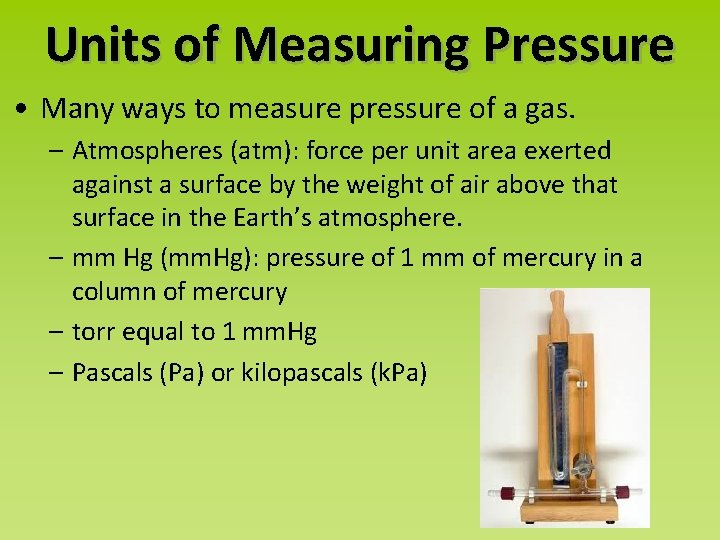Units of Measuring Pressure • Many ways to measure pressure of a gas. – Atmospheres (atm): force per unit area exerted against a surface by the weight of air above that surface in the Earth’s atmosphere. – mm Hg (mm. Hg): pressure of 1 mm of mercury in a column of mercury – torr equal to 1 mm. Hg – Pascals (Pa) or kilopascals (k. Pa)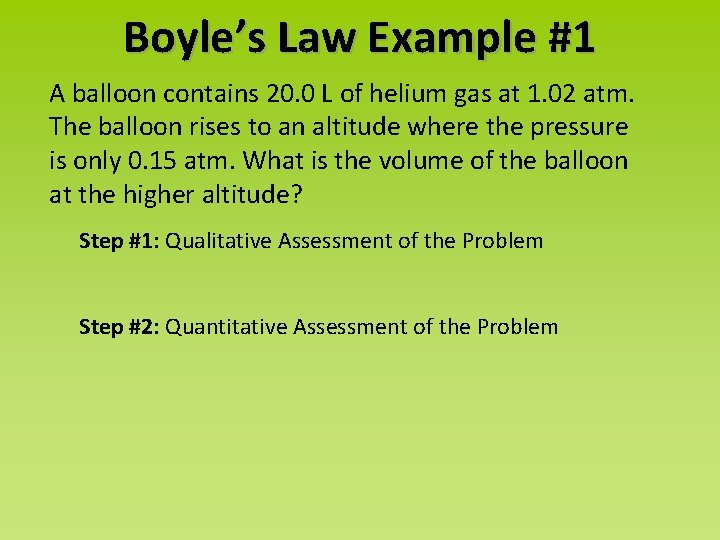Boyle’s Law Example #1 A balloon contains 20. 0 L of helium gas at 1. 02 atm. The balloon rises to an altitude where the pressure is only 0. 15 atm. What is the volume of the balloon at the higher altitude? Step #1: Qualitative Assessment of the Problem Step #2: Quantitative Assessment of the Problem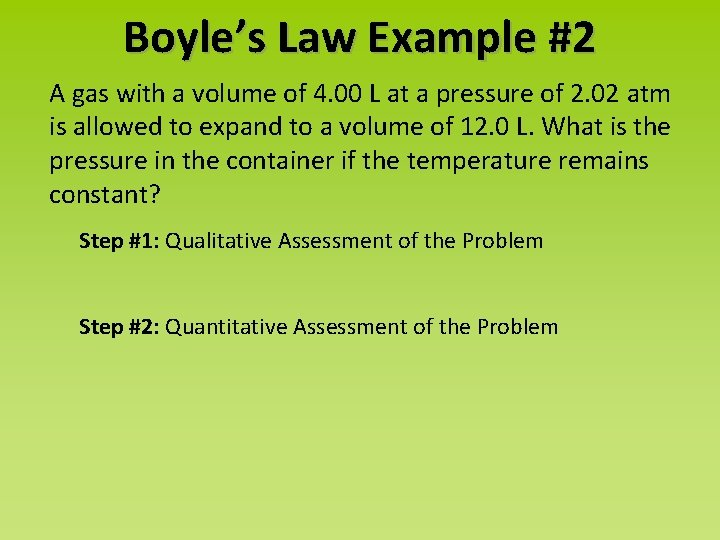Boyle’s Law Example #2 A gas with a volume of 4. 00 L at a pressure of 2. 02 atm is allowed to expand to a volume of 12. 0 L. What is the pressure in the container if the temperature remains constant? Step #1: Qualitative Assessment of the Problem Step #2: Quantitative Assessment of the Problem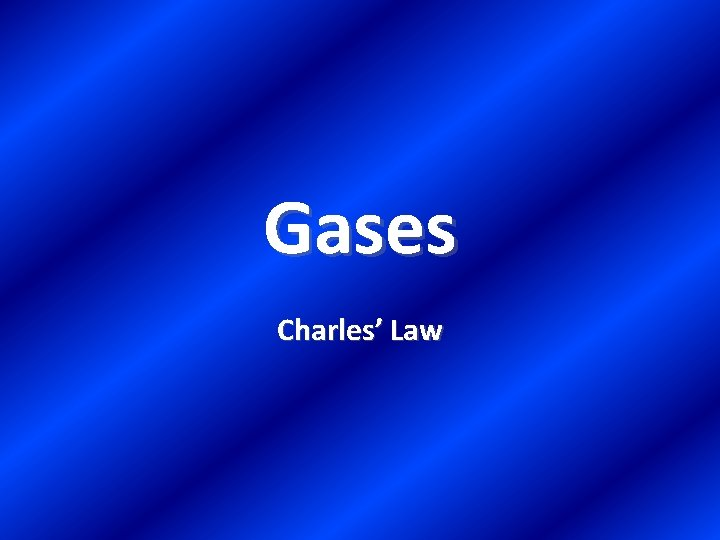Gases Charles’ Law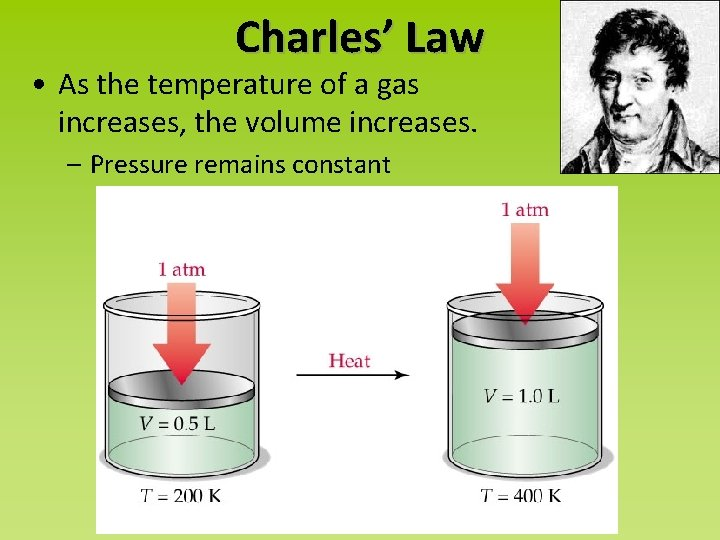Charles’ Law • As the temperature of a gas increases, the volume increases. – Pressure remains constantSymbolic Representation of Charles’ Law As temperature increases, volume increases Volume and temperature are directly proportional Volume (L) Temperature (K) How is this represented in the form of an equation?Measuring Temperature • Fahrenheit (o. F): – based on the freezing and melting point of water • Celsius (o. C): – based on the freezing and melting point of water, but in base 10 • Kelvin (K): – based on the idea that at 0 Kelvin or absolute zero, atoms are NOT in motion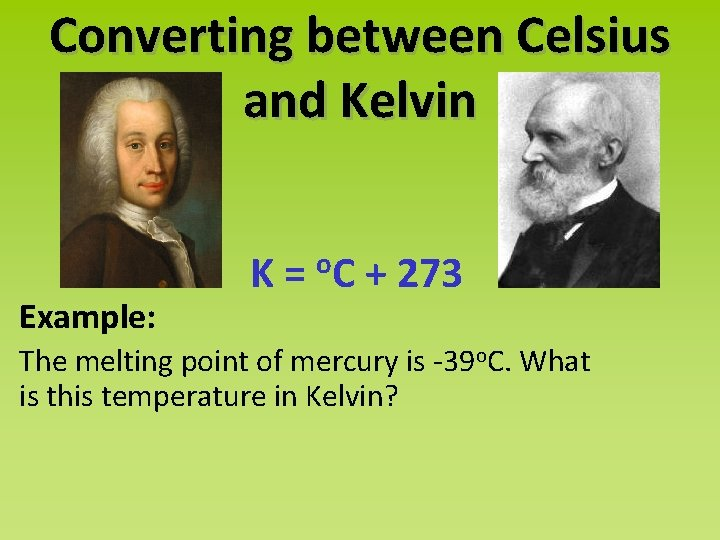Converting between Celsius and Kelvin Example: K = o. C + 273 The melting point of mercury is -39 o. C. What is this temperature in Kelvin?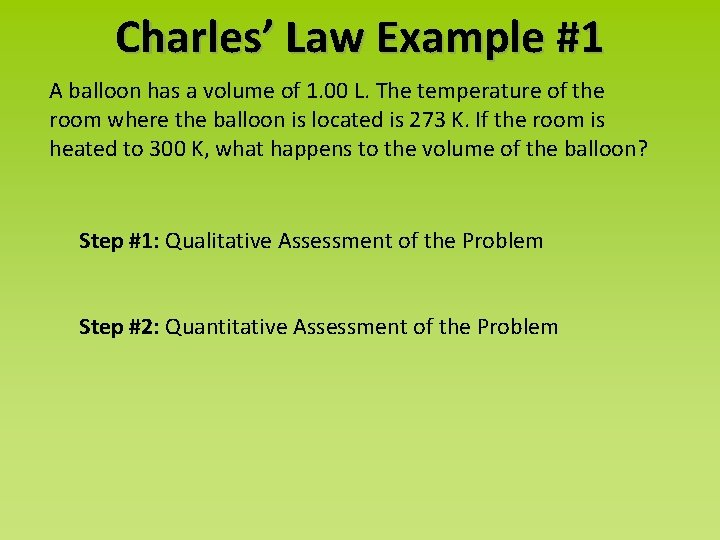Charles’ Law Example #1 A balloon has a volume of 1. 00 L. The temperature of the room where the balloon is located is 273 K. If the room is heated to 300 K, what happens to the volume of the balloon? Step #1: Qualitative Assessment of the Problem Step #2: Quantitative Assessment of the Problem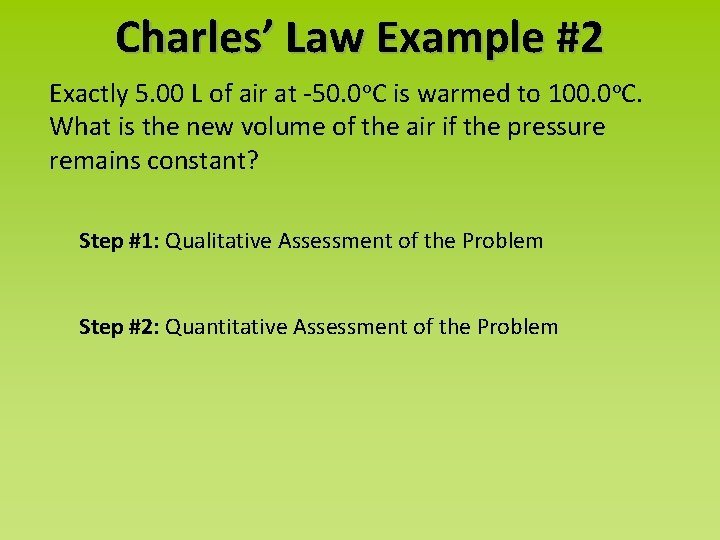Charles’ Law Example #2 Exactly 5. 00 L of air at -50. 0 o. C is warmed to 100. 0 o. C. What is the new volume of the air if the pressure remains constant? Step #1: Qualitative Assessment of the Problem Step #2: Quantitative Assessment of the Problem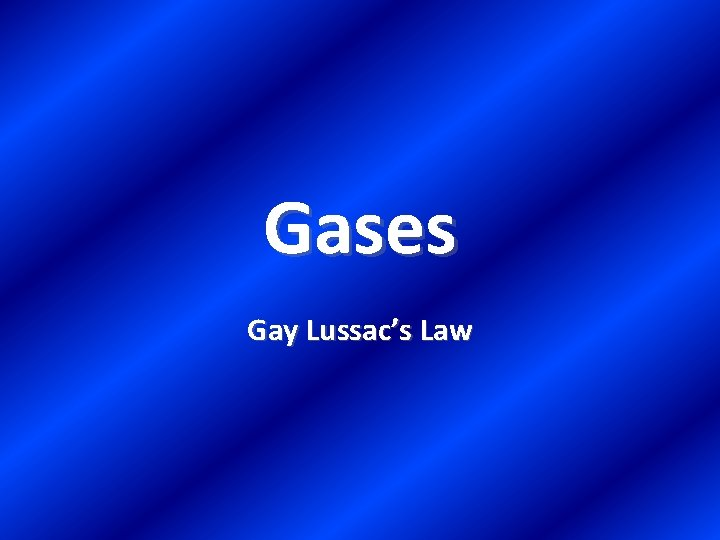Gases Gay Lussac’s LawGay-Lussac’s Law • As the temperature of a gas increases, the pressure increases. – Volume remains constantSymbolic Representation of Gay-Lussac’s Law As temperature increases, pressure increases Pressure and temperature are directly proportional Pressure (atm) Temperature (K) How is this represented in the form of an equation?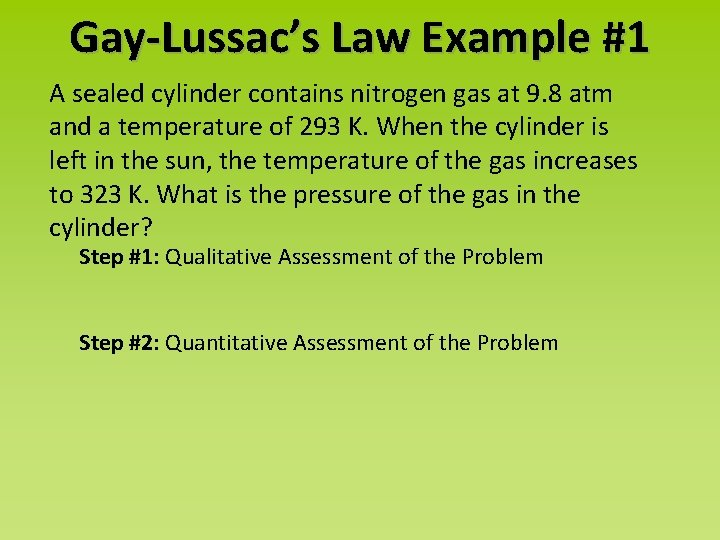Gay-Lussac’s Law Example #1 A sealed cylinder contains nitrogen gas at 9. 8 atm and a temperature of 293 K. When the cylinder is left in the sun, the temperature of the gas increases to 323 K. What is the pressure of the gas in the cylinder? Step #1: Qualitative Assessment of the Problem Step #2: Quantitative Assessment of the ProblemGay-Lussac’s Law Example #2 The pressure in a car tire is 1. 95 atm at 27 o. C. After a long drive, the pressure is 2. 22 atm. What is the temperature of the air in the tire? Assume that the volume is constant. Step #1: Qualitative Assessment of the Problem Step #2: Quantitative Assessment of the Problem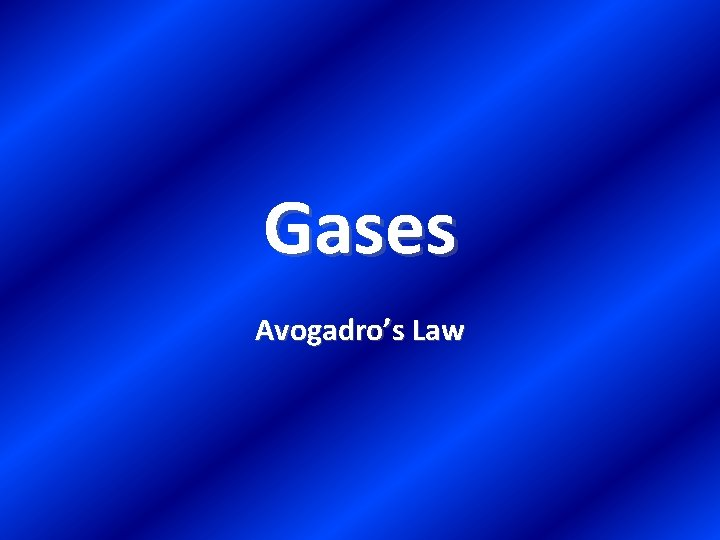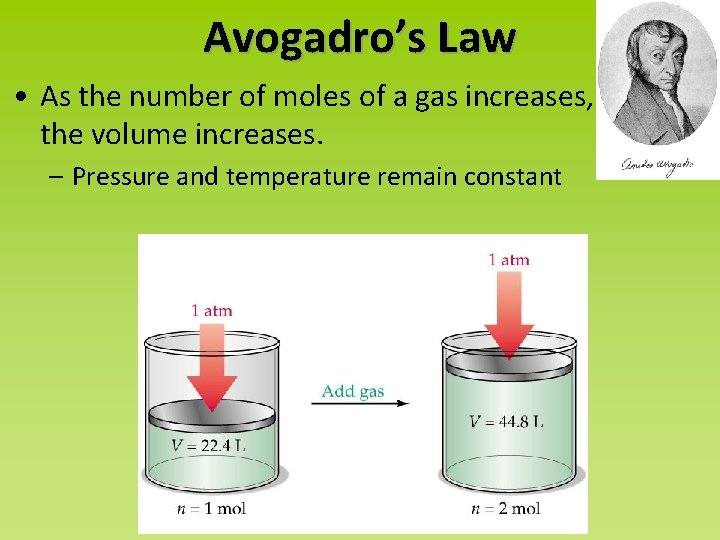Avogadro’s Law • As the number of moles of a gas increases, the volume increases. – Pressure and temperature remain constantSymbolic Representation of Avogadro’s Law As number of moles of gas increases, volume increases Volume (L) Number of moles and volume are directly proportional n (number of moles) How is this represented in the form of an equation?Gases Ideal Gas LawIdeal Gas Law • An ideal gas is defined as one in which all collisions between atoms or molecules are perfectly elastic and in which there are no intermolecular attractive forces. • Think of a gas as a collection of perfectly hard spheres which collide, but which otherwise do not interact with each other. Like billiards!Ideal Gas Law • An ideal gas can be characterized by three variables: absolute pressure (P), volume (V), and absolute temperature (T). • The relationship between them may be deduced from kinetic molecular theory.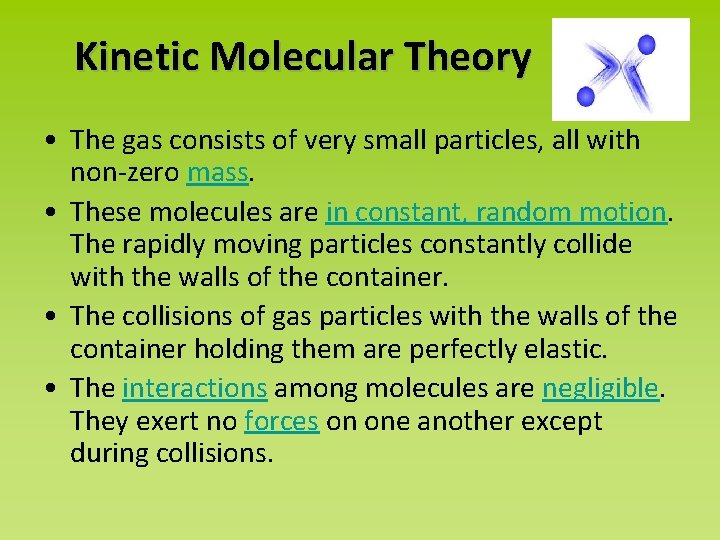Kinetic Molecular Theory • The gas consists of very small particles, all with non-zero mass. • These molecules are in constant, random motion. The rapidly moving particles constantly collide with the walls of the container. • The collisions of gas particles with the walls of the container holding them are perfectly elastic. • The interactions among molecules are negligible. They exert no forces on one another except during collisions.Exceptions to the Ideal Gas Law • at low temperatures (close to 0 K) – gas molecules have less kinetic energy (move around less) so they do attract each other • at high pressures (like many, many atm) – gas molecules are forced closer together so that the volume of the gas molecules becomes significant compared to the volume the gas occupiesSymbolic Representation Ideal Gas Law PV = n. RT • n = number of moles • R = universal gas constant = 0. 0821 L*atm/mol*K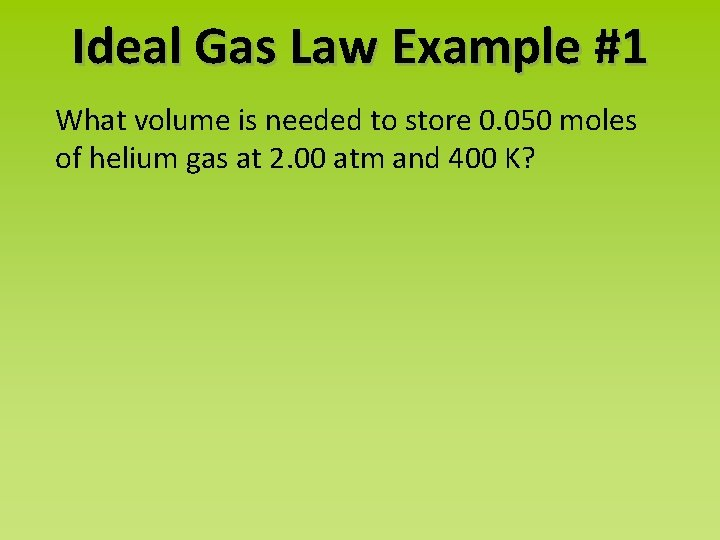Ideal Gas Law Example #1 What volume is needed to store 0. 050 moles of helium gas at 2. 00 atm and 400 K?Ideal Gas Law Example #2 What pressure will be exerted by 20. 16 g of hydrogen gas in a 7. 5 L cylinder at 20 o. C?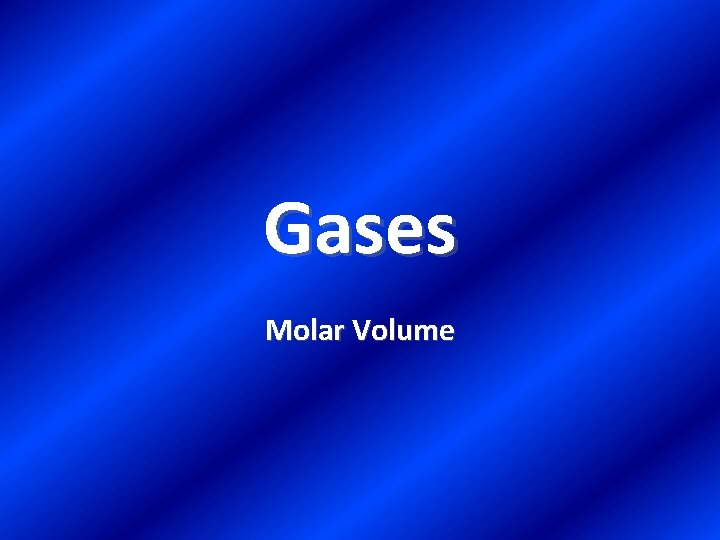Gases Molar VolumeMolar Volume • At standard temp and pressure (STP) 1 mole of any gas occupies 22. 4 L 1 mole = 22. 4 L (use this as a conversion factor) Example: How many liters will 48. 6 grams of CO 2 occupy at STP?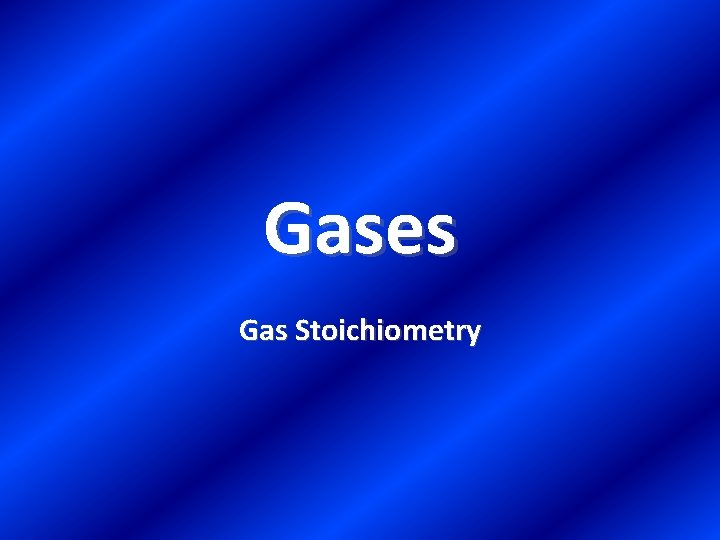Gases Gas Stoichiometry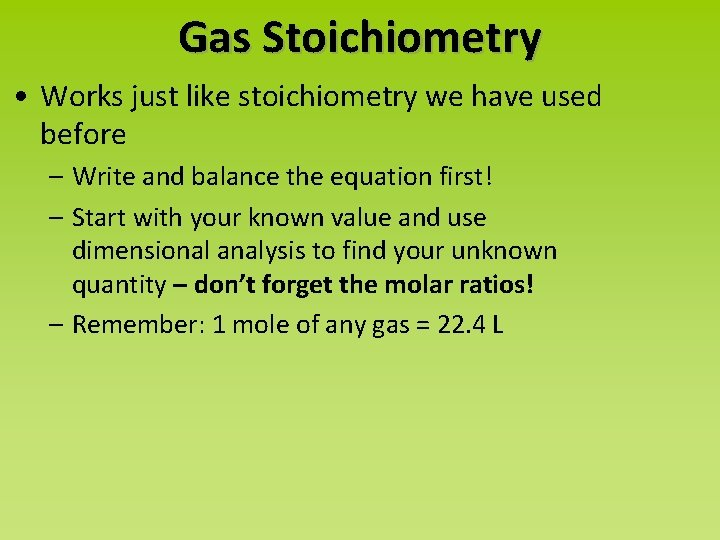Gas Stoichiometry • Works just like stoichiometry we have used before – Write and balance the equation first! – Start with your known value and use dimensional analysis to find your unknown quantity – don’t forget the molar ratios! – Remember: 1 mole of any gas = 22. 4 L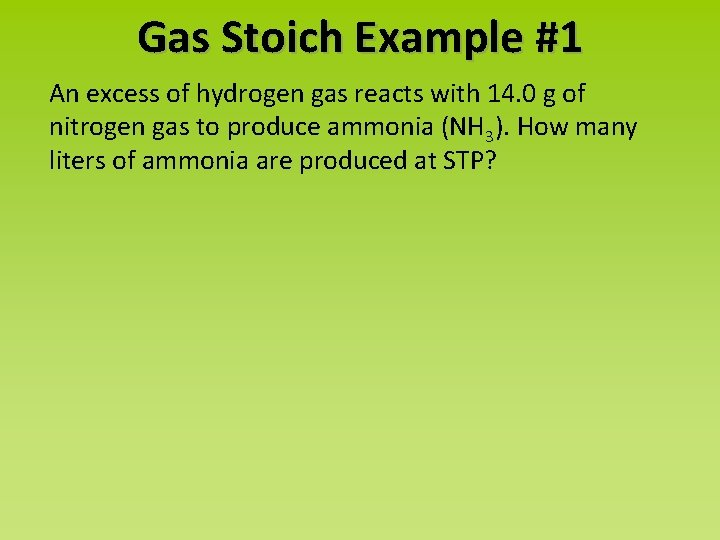Gas Stoich Example #1 An excess of hydrogen gas reacts with 14. 0 g of nitrogen gas to produce ammonia (NH 3). How many liters of ammonia are produced at STP?Gas Stoich Example #2 How many liters of O 2(g) at STP are evolved when 3. 25 g of KNO 3(s) decomposes to KNO 2(s) and O 2 (g) ?Gases Dalton’s Law of Partial PressuresPartial Pressures What do you think the total pressure of the mixture below is?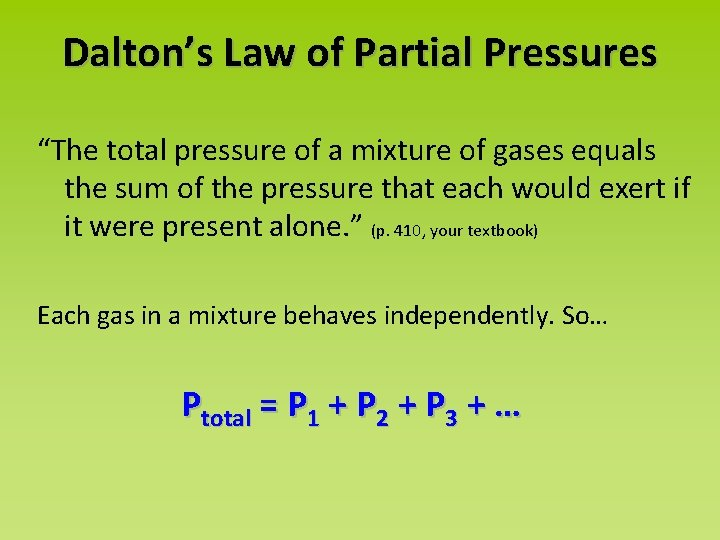Dalton’s Law of Partial Pressures “The total pressure of a mixture of gases equals the sum of the pressure that each would exert if it were present alone. ” (p. 410, your textbook) Each gas in a mixture behaves independently. So… Ptotal = P 1 + P 2 + P 3 + …Mole Fractions & Partial Pressures To determine the partial pressure of a gas…Mole Fraction Example A study of the effects of certain gases on plant growth requires a synthetic atmosphere composed of 1. 50 mol CO 2, 18. 0 mol O 2, and 80. 5 mol Ar. Calculate the partial pressure of O 2 if the total pressure of the this atmosphere is 745 torr.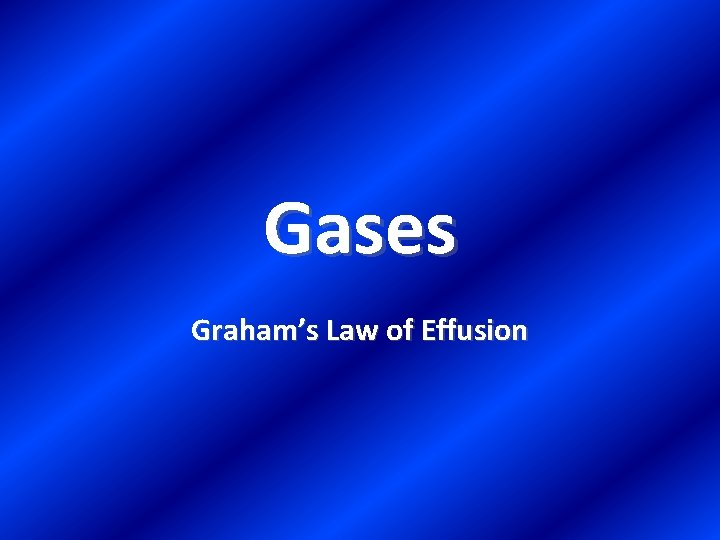Gases Graham’s Law of EffusionEffusion: the escape of gas through a small hole http: //www. chem. ufl. edu/~itl/2041_u 00/lectures/lec_d. html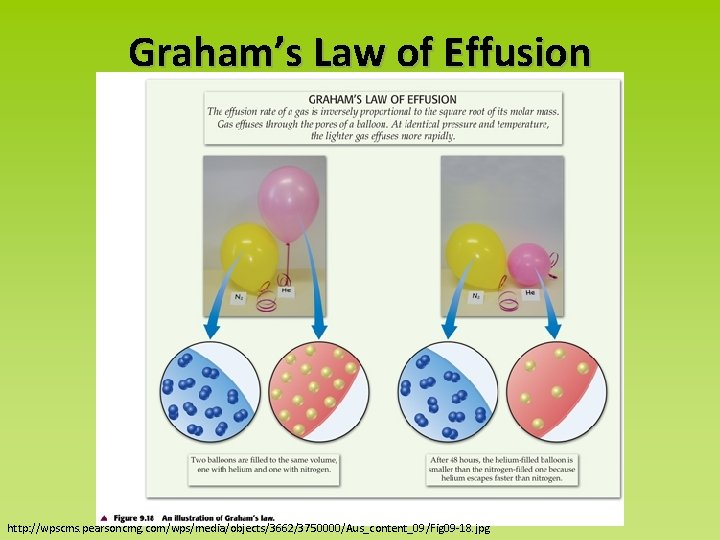Graham’s Law of Effusion http: //wpscms. pearsoncmg. com/wps/media/objects/3662/3750000/Aus_content_09/Fig 09 -18. jpgGraham’s Law of Effusion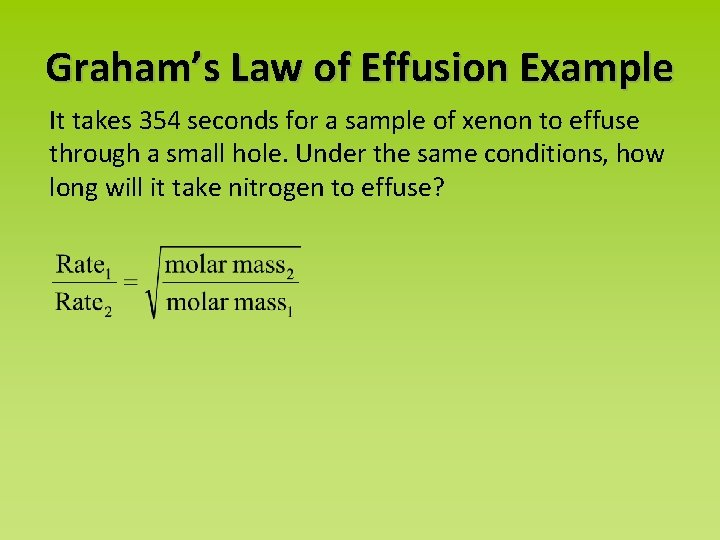Graham’s Law of Effusion Example It takes 354 seconds for a sample of xenon to effuse through a small hole. Under the same conditions, how long will it take nitrogen to effuse?